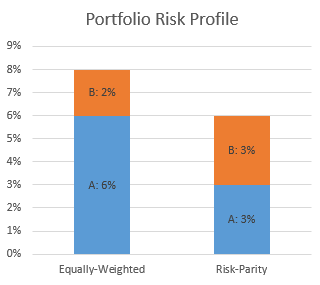# An introduction to Risk Parity

Hello everyone!

In this post, I’d like to start talking again about asset allocation and in particular to introduce you to a relatively new concept in
the field: risk parity. Don’t get me wrong, this approach has been around for quite a while now — I think the first to create a product around this concept were Bridgewater in the 90s — but it is a philosophy which I believe is still not taught frequently enough in finance classes and hence is still widely unknown.# Motivation

I first started to pay attention to risk parity about 5 years ago. In the classic Modern Portfolio Theory (MPT thereafter), the optimization problem has two inputs:

• the covariance matrix of the assets available
• an expected return for each asset considered

Both are difficult to estimate, but researchers tend to agree that estimating covariances is easier than estimating returns.

Nevertheless, a lot of asset managers came up with expected future returns based on “their experience” or on “their view of the market” — there is no real mathematical framework available. This causes a real issue because it has been shown that the mean-variance optimization problem (the one behind MPT) is really sensitive to the expected returns inputs: changing slightly the expected return figures can result in a big optimal allocation change. Therefore, estimating wrong expected returns makes manager select potentially pretty sub-optimal portfolios.Estimating the covariance matrix is not an easy task either. However, there exist frameworks on which managers can rely and estimates they are usually not too far of the reality  (risk tends to be more stable than returns).

What I tried to look for 5 years ago, was an asset allocation method which was independent from the expected returns, as I found them very difficult to estimate accurately. Risk Parity is a perfect example of such method.

# How does it work?

I will try to explain to concept without getting into equations. For those who are interested in the maths, please have a look at Thierry Roncalli’s presentation and paper to start with.

The basic idea is to have a portfolio where risk is diversified as much as possible — ideally perfectly diversified. The remaining challenge is to define how to quantify diversification; defining risk is somewhat arbitrary as you could choose different risk measures (volatility, expected shortfall etc) depending on your preference as an asset manager.

## Quantifying diversification

Let’s assume that we are considering the simplest risk measure, volatility. Once the covariance matrix of all considered assets has been determined, one can then compute the volatility of a portfolio given the weights of each asset. Furthermore, one can also compute how much each asset contributes to the total volatility (i.e. total risk) of the portfolio. In risk parity the goal is to have each asset contributing equally to the total risk.

Let’s consider a portfolio with two assets $A$ and $B$ with 50% of weight for each asset (so-called equally-weighted portfolio) and let’s assume the total volatility is 8%. By computing the contribution and assuming $A$ is more volatile than $B$, then you could see that $A$ contributes by 6% and $B$ by 2% only (numbers here are arbitrary and taken for the sake of the example).According to our diversification measure, the portfolio is not very well balanced as it is much more exposed to $A$.

## Finding risk-parity

In his paper, Roncalli shows how one can implement an optimization method to find the portfolio where both $A$ and $B$ would equally contribute to the portfolio’s total volatility. Since I want to keep this post fairly non-technical I voluntarily want to skip the details of the methodology. Let’s assume we are provided with an optimizer which gives us the risk-parity portfolio. It would look like something with 70% weight on $B$ and 30% on $A$ and which would result in a total volatility of about 3%.This time, our portfolio is optimally balanced as it is equally exposed to both assets from a risk point of view. Note that the total volatility of the risk-parity portfolio cannot be chosen, it is purely a result of the optimization.

There is much more to say on the properties of risk-parity, and I will write more about this in later posts.